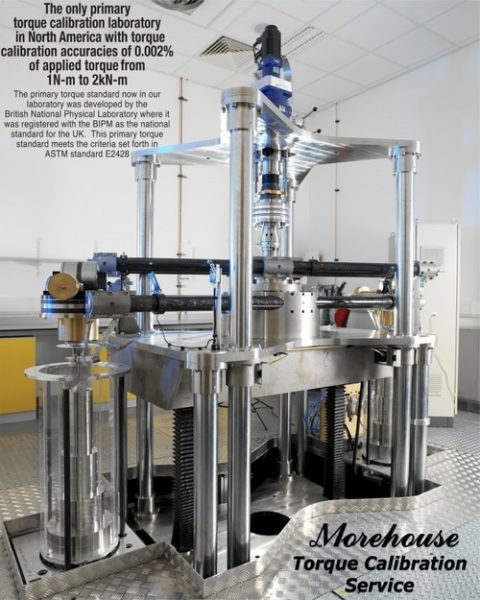Torque Formulas and Conversions

Torque ExplainedIn the simplest sense Torque = Force * Length

Torque is derived and traceable to SI units of length,  mass,  and time.

The meter is the length of the path traveled by light in vacuum during a time interval of 1/299 792 458 of a second.

The kilogram is the unit of mass; it is equal to the mass of the international prototype of the kilogram.

The second is the duration of 9 192 631 770 periods of the radiation corresponding to the transition between the two hyper-fine levels of the ground state of the cesium 133 atom.— Torque , also called  moment  or  moment of force  is the tendency of a force to rotate an object about an axis, fulcrum, or pivot. Just as a force is a push or a pull, a torque can be thought of as a twist.

To convert from one unit of torque to another, requires knowing the appropriate conversions.

Torque =  Force  x Length

Force Conversion Factors

newton (N) to lbf = MULTIPLY BY  0.2248089431

newton (N) to kgf = MULTIPLY BY  0.1019716212978

newton (N) to ozf = MULTIPLY BY 3.59694309

lbf to newton (N) = MULTIPLY BY 4.4482216152605

lbf to KGF = MULTIPLY BY 0.45359237

lbf to ozf  = MULTIPLY BY 16

kgf to lbf = MULTIPLY BY 2.20462262

kgf to newton (N) = MULTIPLY BY 9.80665

Kgf to ozf = MULTIPLY BY 35.27396

ozf to newton (N) = MULTIPLY BY 0.2780139

ozf to lbf = MULTIPLY BY  0.0625

ozf to kgf = MULTIPLY BY 0.0283495231

Torque =   Force x  Length

Length Conversion Factors

METER (m) to FEET (ft) = MULTIPLY BY  3.2808399

METER (m) to INCHES (in) = MULTIPLY BY 39.3700787

FEET (ft) to METERS (m) = MULTIPLY BY  0.3048

FEET (ft) to INCHES (in) = MULTIPLY BY 12

INCHES (in) to FEET (ft)= MULTIPLY BY 0.0833333333

INCHES (in) to METERS (m) = MULTIPLY BY 0.0254

Examples

Force  1N = 0.2248089431 lbf         Length 1m = 3.280839895 ft

—1 N-m = 0.2248089431 x 3.280839895 = 0 .7375621489  ft-lbf

Force  1N = 0.2248089431 lbf         Length 1m = 39.3700787    in

—1 N-m = 0.2248089431 x   39.3700787=  8.50745782  in-lbf

Everything we do, we believe in changing how people think about force and torque calibration.  Morehouse believes in thinking differently about force and torque calibration and equipment.  We challenge the "just calibrate it" mentality by educating our customers on what matters, what causes significant errors, and focus on reducing them.  Morehouse makes our products simple to use and user-friendly.  And we happen to make great force equipment and provide unparalleled calibration services.

Wanna do business with a company that focuses on what matters most?  Email us at info@mhforce.com.

• We're committed to your privacy. Morehouse Instrument Company uses the information you provide to us to contact you about our relevant content, products, and services. You may unsubscribe from these communications at any time. For more information, check out our Privacy Policy.